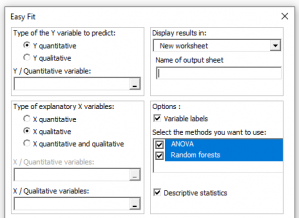# Easy Fit / Easy Predict

Use this feature to test and compare different predictive models for the same dataset. Available in Excel using the XLSTAT software.Easy Fit allows to test and compare different predictive models for the same dataset. Depending on the type of the dependent and explanatory variables (quantitative or qualitative), various models are proposed. The Easy Predict function can be then used to make predictions using the previously fitted models.

## Options for the Easy Fit /  Easy Predict fuction in XLSTAT

### Available regression models

Easy Fit offers the following regression models:

• If the X explanatory variables are only quantitative:
• If the X explanatory variables are only qualitative:
• If some X explanatory variables are quantitative and other qualitative:

### Available classification models

Easy Fit offers the following classification models (you can click on the different methods to access the associated help document):

• If the X explanatory variables are only quantitative:
• If the X explanatory variables are only qualitative:
• If some X explanatory variables are quantitative and other qualitative:

## Results for the Easy Fit /  Easy Predict fuction in XLSTAT

Descriptive statistics: The tables of descriptive statistics show basic statistics for all the selected variables such as the number of observations, missing values, the number of non-missing values, the mean and the standard deviation (unbiased) are displayed for the quantitative variables. For qualitative variables the names of the various categories are displayed together with their respective frequencies.

Summary table: This table presents the adjustment coefficients for assessing the quality of the model. Missclassification rate is used for classifications and the mean squared errors for regression models. Adjustment coefficients are calculated on the validation sample.

Summary results by method: For each method the most important results of the method are displayed. For a better understanding of the various results, you can access the help section of the related methods.### analyze your data with xlstat

14-day free trial

Included in